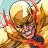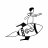# After-Hours Relative Volume Indicator for ThinkorSwim#### BenTen

Staff
VIP
Someone on Github recently shared this indicator called After Hours Relative Price Movement.

Tracks the price difference from afterhour volume relative to yesterday's close. This was created to visually confirm if certain stock prices were primarily driven from afterhour movements over longer periods of time.### thinkScript Code

Code:
``````# AfterHoursRelativePriceMovement tracks the price difference from afterhour volume relative to yesterday's close

# This was created so I could eyeball if certain stock prices were primarily driven from afterhour movements over longer periods of time
# Can be overlaid with AddMarketHoursUTC to visually see correlation from international market open/closes

# Inputting Market Hours is required due to thinkscript's date function limitations
input marketOpen = 0930;
input marketClose = 1600;

# AFAIK there is no better way to do this due to SecondsTillTime rolling over after midnight, lack of real date math, and the mish-mash of UTC/EST time handling from thinkscript
def isClosedMorning = if SecondsTillTime(marketOpen) > 0 then yes else no;
def isClosedNight = if SecondsTillTime(marketClose) < 0 then yes else no;

def change = if !isClosedMorning AND !isClosedNight then 0 else change + (hl2 - hl2);

plot yesterdayPrice = 0;

plot priceDifference = if change != 0 then change else Double.NaN;
priceDifference.AssignValueColor(if priceDifference > 0 then Color.UPTICK else if priceDifference < 0 then Color.DOWNTICK else Color.GRAY);

# This is to visually encapsulate a week because weekend price movements can be funky
def isStartOfWeek = if GetDayOfWeek(GetYYYYMMDD()) == 1 then yes else no;
def isEndOfWeek = if GetDayOfWeek(GetYYYYMMDD()) == 5 then yes else no;

AddVerticalLine(isStartOfWeek AND SecondsTillTime(marketOpen) == 0, "Monday Open", Color.GREEN);
AddVerticalLine(isEndOfWeek AND SecondsTillTime(marketClose) == 0, "Friday Close", Color.RED);``````
Credit:

•RickAns
R

#### Robby Luca

##### New member
Hello,

I am trying to get the Relative Volume during afterhours/premarket but I have been unsuccessful.
What I am looking for exactly is a script that will give me the current day volume (including premarket) divided by the Daily Average Volume of last 60 days.

My initial script:

input length = 60;
def avgvol = average(volume, length);
plot rv = round(volume/avgvol,2);

The issue I encounter is that if I put Day Timeframe then during the extended hours, it does not calculate the current volume (during premarket).
Then if I put 1m Timeframe then it will be the average volume of last 60 minutes.

So I attempted using aggregation:

input length = 60;
def agg = aggregationPeriod.DAY;
def avgvol = average(volume(period = agg), length);
plot rv = round(volume(period = agg)/avgvol,2);

No success. Any suggestions? Thank you!

R

#### Robby Luca

##### New member
Not what I’m asking for. But thank you. There’s no volume in that code if I’m understanding it correctly. It’s all about price.

R

#### RickAns

##### Member
VIP
Robby, the poster Sonny has a few threads dealing with Relative Volume and premarket happenings. The code you posted is very similar to some in his watch list thread https://usethinkscript.com/threads/color-coded-watchlist-columns-for-thinkorswim.2977/ . If you have not seen it then it might interest you.

Similiar bit:

RV1
#Relative Volume
def x = Average(volume, 60);
def v = volume;
plot r = Round((v/x),1);
r.assignValueColor(if r >= 20 then color.CYAN else if r>=5 then createcolor(255,153,153) else createcolor(0,215,0));

•BenTen
R

#### Robby Luca

##### New member
That's the exact one I posted and used but like I mentioned does not work in premarket. Will check out the thread.

M

#### malone1020

##### New member
VIP
I have ended up with three different Relative Volume Watchlist codes and am now confused how these differ. Anyone willing to help me decipher these three? Is it just as simple as 1 = 60 days benchmark, 2 = 50 days and 3 = 10 days?

Thank you!

1. -------------------------------
Code:
``````## OneNote Archive Name: Relative Volume to past days Average Relative Volume _v02_Mobius
## Suggested Tos Name using JQ naming convention: RelVolumeVsAvgRelVolume_v02_Mobius
## OneNote Section: Volume
## Archive Date: 02.10.2019
## Provenance: Posted by Mobius himself in the Lounge on 02.05.2019

# Relative Volume to past days Average Relative Volume
# Mobius
# Chat Room Request 01.23.2017

input length = 60;

def v = volume(period = AggregationPeriod.Day);
def active = if secondsFromTime(0930) > 0 and
secondsTillTime(1600) >= 0
then 1
else 0;
/ GetAggregationPeriod());
def newDay = GetDay() != GetDay();
def DayVol = if newDay
then v
else DayVol + v;
def avgVolume = (fold day = 0 to length
with s
do s + GetValue(DayVol, day * Bars, (length - 1) * Bars)) / length;

plot R = DayVol / avgVolume * 100;
R.SetPaintingStrategy(PaintingStrategy.Histogram);
R.AssignValueColor(if R > 100
then color.green
else color.red);
# End Code``````
2. ------------------------------------------

Code:
``````# rvol watchlist column
# 10-31-2019

def volumeAvgPeriodDays = 50;
def volumeAvg = (fold index=1 to volumeAvgPeriodDays+1 with acc=1 do acc+volume(period="DAY")[index]) / volumeAvgPeriodDays;

def volumeToday = volume(period="DAY");

plot a = Round((volumeToday / volumeAvg), 2);``````
3. ---------------------------------------------------------------------------------

Code:
``````input STime = 0930 ; #hint STime: Start of normal trading hours
input ETime = 1600 ; #hint ETime: End of normal trading hours
input thold = 1.5 ; #hint told: Threshold for color break
def bpd = 26 ; # Bars per day during active trading hours
def daycnt = 10; # Number of days

def IsActive = if secondsTillTime(ETime) > 0 and
secondsFromTime(STime) >= 0
then 1
else 0;

# This is the cumulative volume for the current day between STime and ETime
def cumVolume = if IsActive and !IsActive
then volume
else if IsActive then cumVolume + volume
else cumVolume;

# This is the average cumulative volume over the same time period from the previous 10 days
def cumVolumePrev = if IsActive and !IsActive
then (volume[(bpd*1)+1] + volume[(bpd*2)+1] + volume[(bpd*3)+1] + volume[(bpd*4)+1] + volume[(bpd*5)+1] + volume[(bpd*6)+1] + volume[(bpd*7)+1] + volume[(bpd*8)+1] + volume[(bpd*9)+1] + volume[(bpd*10)+1] ) / daycnt
else if IsActive then cumVolumePrev + (volume[(bpd*1)+1] + volume[(bpd*2)+1] + volume[(bpd*3)+1] + volume[(bpd*4)+1] + volume[(bpd*5)+1] + volume[(bpd*6)+1] + volume[(bpd*7)+1] + volume[(bpd*8)+1] + volume[(bpd*9)+1] + volume[(bpd*10)+1] ) / daycnt
else cumVolumePrev;

plot RelVol = cumVolume / cumVolumePrev ;

RelVol.assignValueColor(if RelVol > thold then CreateColor(0,128,0) else CreateColor(128,0,0) );``````Check/Force Turning Off Extended Hours Questions 2Is it possible to show lunch hours on my chart? Questions 9